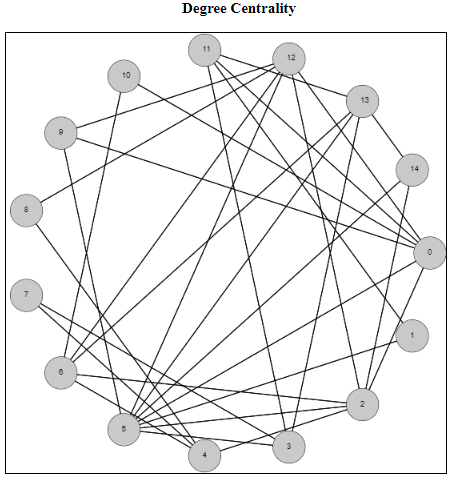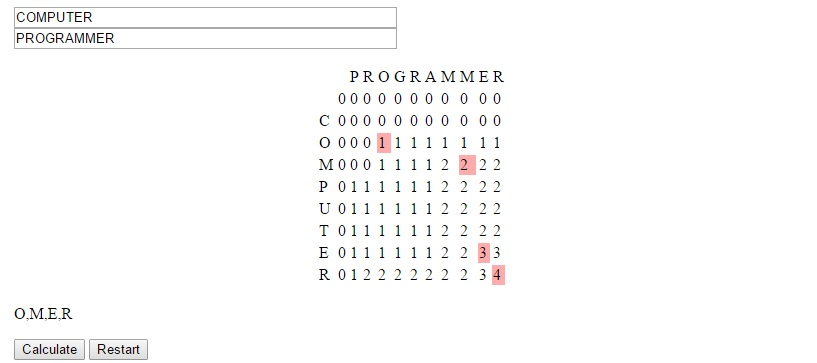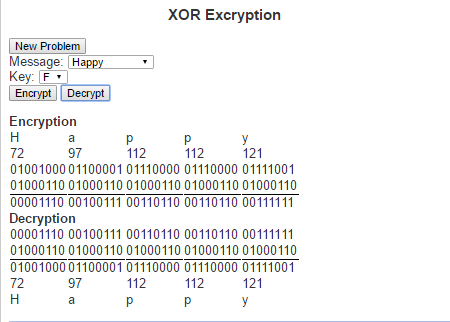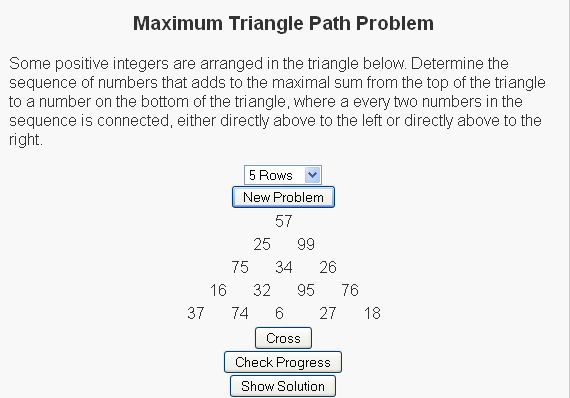# Pascal’s Triangle

I had a recent conversation with a friend who asked me “what makes number theory interesting?”. I loved the question, mainly because it gave me an opportunity to talk about math in a positive manner. More importantly though, it was an opportunity to talk about one of my favorite courses in mathematics (along with discrete mathematics and set theory). As much as the current day seems to focus on joining Number Theory with Cryptography, when I answered this question I wanted to make sure I didn’t go that route. Numbers are beautiful in their own right, and one of the things about Number Theory that was so interesting was simply the ability to look at all the different questions and patterns and properties of numbers discovered.

To answer this question, I started listing numbers to see if she noticed a pattern, but I did it with a “picture”.

.
..

….
…..
……

• How many dots will go on the next line
and
• After each line how many dots have been drawn in total?

Dots# on this line# in total
.11
..23
36
….410
…..515

There were a lot of directions I could have taken this conversation next, but I decided to stay in the realm of triangles and discuss Pascal’s triangle. This is a triangle that begins with a 1 on the first row and each number on the rows beneath is the sum of the two cells above it, assuming that cells not present have a value of zero.

So the first five rows of this triangle are

1
11
121
1331
14641

This is an interesting and beautiful triangle because of just the number of patterns you can see in it.

• Obviously there are ones on the outside cells of the triangle.
• One layer in, we get what are called the Natural or Counting numbers (1, 2, 3, 4, 5, …) .
• One layer in, we can start to see the list of numbers that I was showing my friend (1, 3, 6, 10, 15, …).

There are several other properties of this triangle and I wanted to allow users to begin to see them, so I wrote a script highlighting some of these patterns.

# Degree Centrality of a GraphI wanted to spend some time on centrality measures of a graph. These are measurements of how important each node (or edge) is to the overall graph. But how do we define, or determine, importance? There is no unique way to answer this question, so there are varying metrics for measuring centrality. Which one you choose depends on several factors including how many other nodes of the graph are included, as well as the run time of the metrics you’re considering.

I have just published a script focusing on the degree centrality metric. The degree centrality metric is called a “walk metric” because it determines how important a node is by how many other nodes that can be reached by walks of up to a certain length. Lets look at the definition of the degree of a node to see if we can understand why it is called a walk metric.

In an undirected graph G = (V, E), the degree of a node u [in] V is the |{v | (u, v) [in] E}|. This is the size of the set of nodes that are connected to node u via a single edge. Another way of describing a single edge is a walk of length one. So the degree metric measures the importance of a node by the number of unique walks of length one.

The normalized degree centrality of a node v in a graph G = (V,E) measures how many nodes are connected to the node v, compared to the maximum possible number of edges that can be connected to this node. Because we are dealing with simple undirected graphs (at most a single edge between any two distinct vertices), this maximum possible number will always be |V – 1|. So the normalized degree can be calculated by dividing the degree of the node (the number of nodes it is connected to) by |V – 1|.

So for the example above, the node 0 has degree 6 because it is connected to nodes 2, 5, 9, 10, 11, and 12. There are 15 total nodes in this graph, so to calculate the normalized degree centrality of the node 0, it will be 6 / 14, which rounds to 0.428571.

To see more examples and to help answer questions, check out the script in my examples section on degree centrality

# Longest Common Subsequence

Suppose you and I each had an ordered list of items and we were interested in comparing how similar those lists are. One calculation we can perform on these two strings is the Longest Common Subsequence. A sequence X is an ordered list of elements <x1, …, xn>. A subsequence Z is another sequence where (1) Each element of Z is also an element of X and (2) The elements of Z occur in the same order (in Z) as they do in X.

Note that we do not say that the elements of Z need to be a continuous block of elements. If this were true we would be defining a substring. So as an example, suppose we have as an initial string,
X = C, O, M, P, U, T, E, R.
Then the following are all subsequences:
Z1 = C, M, U, T, R
Z2 = C, O, M, P
Z3 = U, T, E, R
Z4 = O, P, T, E

I will note that Z2 and Z3 are also substrings since they contain continuous sets of characters.

The length of a substring is simply the number of characters it contains. So X has length 8, Z1 has length 5, Z2, Z3 and Z4 have length 4.

Suppose now that we had a second string, Y = P, R, O, G, R, A, M, M, E, R and are interested in the longest common subsequence between the two. We can do that by observing that there is a bit of recursion going on with this question. What I mean by that is that asking the question of “What is the longest common subsequence between X and Y” is the same as asking “What is the longest common subsequence between X and Y once we have seen 8 characters of X and 10 characters of Y”

There are three possible ways to answer this question.

If X<sub>8</sub> is not equal to Y<sub>10</sub>, then the answer to this will be the same as the maximum of the pair X<sub>7</sub>, Y<sub>10</sub> and the pair X<sub>8</sub>, Y<sub>9</sub>.
If we reach a situation where we reach the beginning of either string, we are forced to answer 0 to that question.

Then the function has the following look:

LCS(Xi, Yj) =
 0, if i is 0 or j is 0 1 + LCS(Xi-1, Yj-1) if Xi equals Yj max(LCS(Xi-1, Yj), LCS(Xi, Yj-1))

Below is a table showing how we would solve the problem mentioned.The strategy used to devise this concept is called dynamic programming. It is useful we can solve larger problems by solving overlapping subproblems, as was the case here. In this situation we generally can store the data in a table form and avoid re-solving subproblems for which many larger problems will be dependent.

You can see this algorithm in action at LEARNINGlover.com: Longest Common Subsequece. Let me know what you think.

# Lets Learn About XOR EncryptionOne of the more common things about this generation is the constant desire to write up (type) their thoughts. So many of the conversations from my high school days were long lasting, but quickly forgotten. Today’s generation is much more likely to blog, tweet, write status updates or simply open up a notepad file and write up their thoughts after such a conversation.

When we feel that our thoughts are not ready for public eyes (maybe you want to run your idea by the Patent and Trademark Office before speaking about it) we may seek some form of security to ensure that they stay private. An old fashioned way of doing this was to write in a diary and enclosed it within a lock and key. The mathematical field of encryption also tries to grant privacy by encoding messages so that only people with the necessary information can read them.

The type of encryption I want to speak about today is called XOR encryption. It is based on the logical operation called “exclusive or” (hence the name XOR). The exclusive or operation is true between two logical statements if exactly one of the two statements is true, but not both statement. This can be represented with the following truth table

 Input 1 Input2 XOR Result T T F T F T F T T F F F

XOR Encryption is particularly useful in this day and age because we every character we type is understood by the computer as a sequence of zeros and ones. The current standard encoding that is used is Unicode (also known as UTF-8). Under this encoding the letter ‘a’ is represented as the binary string ‘01100001’. Similarly every letter, number and special character can be represented as its own binary string. These binary strings are just an assignment of numbers to these characters so that we can to help represent them in the computer. The numbers can the be thought of in base 10, which is how we generally think about numbers, or in base 2 which is how computers generally work with numbers (or a number of other ways). The way we would use these binary strings in encoding is first by translating a text from human-readable text to machine readable text via its binary string. For example, the word “Invincible”, we would get the following binary strings:

 Letter Unicode in base 10 Unicode in base 2 I 73 01001001 n 110 01101110 v 118 01110110 i 105 01101001 n 110 01101110 c 99 01100011 i 105 01101001 b 98 01100010 l 108 01101100 e 101 01100101

To encrypt the message we need a key to encode the message and will simply perform an XOR operation on the key and every character in the string. Similarly, do decrypt the message we perform XOR operation on the key and every character in the encoded message. This means that the key (much like a normal key to a diary) must be kept private and only those whom the message is to be shared between have access to it.

Here is a link to the script where you can check out XOR Encrpytio. Try it out and let me know what you think.

# Triangle Sum Puzzle

This is probably a consequence of being a mathematician, but I have always enjoyed number puzzles. I think that there is a general simplicity and universality in numbers that are not present in things like word puzzles, where the ability to reach a solution can be limited to the vocabulary of the user.

The fact that these are puzzles and not simply homework exercises also helps because we often find people sharing difficulties and successes stories over the water cooler or at the lunch table. The fact that many of these math puzzles can teach some of the same concepts as homework problems (in a more fun and inclusive way) is generally lost on the user as their primary interest is generally on solving the puzzle in front of them, or sometimes solving the more general form of the puzzle.

Today’s post is about a puzzle that was originally shared with me over a lunch table by a friend who thought it was an interesting problem and asked what I thought about it. I didn’t give the puzzle much further thought (he had correctly solved the puzzle) until I saw it again in “Algorithmic Puzzles” by Anany Levitin and Maria Levitin. It was then that I thought about the more general form of the puzzle, derived a solution for the problem, and decided to code it up as a script for my site.

Below is a link to the puzzle:We have a set of random numbers arranged in a triangle and the question is to find the path of maximum sum from the root node (the top node) to the base (one of the nodes on the bottom row) with the rules that
(1) Exactly one number must be selected from each row
(2) A number can only be selected from a row if (a) it is the root node or (b) one of the two nodes above it has been selected.

For the sample
So for the sample problem in the picture, the maximal path would go through nodes 57, 99, 34, 95, and 27.

For more of these puzzles check out the script I write here and be sure to let me know what you think.